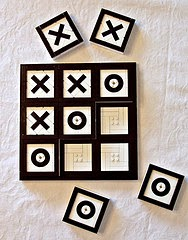Programming Learning: Tic Tac Toe Game Tutorial for C++ - ByteScout
• Home
• /
• Blog
• /
• Programming Learning: Tic Tac Toe Game Tutorial for C++

# Programming Learning: Tic Tac Toe Game Tutorial for C++

## What’s a tic-tac-toe game?

Since many of you already know, tic-tac-toe is a 2 player game which is played on a grid of 3 squares to 3 squares. The main objective of this game is to score three’s in a row. This game also goes by the names “O’s and X’s” or “Noughts and crosses (British language)” in some languages. It is traditionally played on a piece of paper but now you can even download tic-tac-toe applications and play it on your phone.

## History behind tic-tac-toe

Around the 1st century B.C, this game was first introduced during the Roman Empire. It didn’t go by its current name but rather was called “three pebbles at a time”. If you search over the internet, you will even find images of the game’s grid which were found drawn on many Roman ruins. Not only Roman but Egyptian ruins also bear witness to such chalking.

The game was first called ‘tic-tac-toe’ in 1884 but was considered as a children’s game.

## How to play?

Now that we are aware of the game and its history, let’s get to the rules and regulations considered while playing this game.

You might already know to play tic-tac-toe since you think it’s a very easy and simple game, right? Well, that’s where most people are wrong. If you really get your mind around the game, you will find out that it is not a simple as you thought it would be.

Let’s take a look at the rules for tic-tac-toe:

•         The game is played on a 3 by 3 square grid.
•         It’s a 2 player game.
•         One player picks the ‘X’ mark and the other is ‘O’ mark.
•         You only get one turn for one mark to put in empty squares.
•         Whichever player gets 3 of their marks in a row, wins.
•         The marks can be made diagonally, vertically or horizontally.
•         When all of the 9 squares are filled up, the game is over.
•         If neither of the two players is successful in having 3 marks in a row, the game ends in a tie.

## Secret Strategies to win Tic-tac-toe

Tic-tac-toe is a very mindful game. You have to be aware of every move of your opponent and even figure out which move you will make next.

To beat your opponent, be it a person or a computer even, you need to plan out a strategy.

You first need to figure out how you can make those three X’s in a row and stop your opponent from winning as well.

Watch your opponent’s every move. For example, if they put two O’s in a row, then you MUST put an X in the next square to those consecutive O’s or you’ll lose.

In short, you must be very vigilant while making your moves. This is the only way you’ll never lose a game of tic-tac-toe. If you’ll not win then at least you will have a tie. GOOD LUCK!Here’s a beginner project: Tic Tac Toe. The code below contains the solution needed to create a tic tac toe game.

``````   #include <iostream>
using namespace std;``````

char square = {‘o’,’1′,’2′,’3′,’4′,’5′,’6′,’7′,’8′,’9′};

int checkwin();
void board();

int main()
{
int player = 1,i,choice;

char mark;
do
{
board();
player=(player%2)?1:2;

cout << “Player ” << player << “, enter a number: “;
cin >> choice;

mark=(player == 1) ? ‘X’ : ‘O’;

if (choice == 1 && square == ‘1’)

square = mark;
else if (choice == 2 && square == ‘2’)

square = mark;
else if (choice == 3 && square == ‘3’)

square = mark;
else if (choice == 4 && square == ‘4’)

square = mark;
else if (choice == 5 && square == ‘5’)

square = mark;
else if (choice == 6 && square == ‘6’)

square = mark;
else if (choice == 7 && square == ‘7’)

square = mark;
else if (choice == 8 && square == ‘8’)

square = mark;
else if (choice == 9 && square == ‘9’)

square = mark;
else
{
cout<<“Invalid move “;

player–;
cin.ignore();
cin.get();
}
i=checkwin();

player++;
}while(i==-1);
board();
if(i==1)

cout<<“==>aPlayer “<<–player<<” win “;
else
cout<<“==>aGame draw”;

cin.ignore();
cin.get();
return 0;
}

/*******************************************************************
FUNCTION TO DRAW BOARD OF TIC TAC TOE WITH PLAYERS MARK
********************************************************************/

void board()
{
system(“cls”);
cout << “nntTic Tac Toenn”;

cout << “Player 1 (X) – Player 2 (O)” << endl << endl;
cout << endl;

cout << ” | | ” << endl;
cout << ” ” << square << ” | ” << square << ” | ” << square << endl;

cout << “_____|_____|_____” << endl;
cout << ” | | ” << endl;

cout << ” ” << square << ” | ” << square << ” | ” << square << endl;

cout << “_____|_____|_____” << endl;
cout << ” | | ” << endl;

cout << ” ” << square << ” | ” << square << ” | ” << square << endl;

cout << ” | | ” << endl << endl;
}

photo credit: Tic-Tac-Toe via photopin (license)